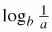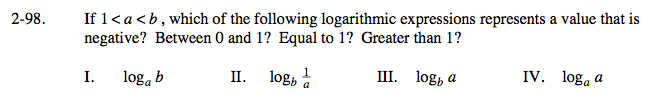### Home > CALC > Chapter Ch2 > Lesson 2.2.4 > Problem2-98

2-98.
1. If 1 < a < b, which of the following logarithmic expressions represents a value that is negative? Between 0 and 1? Equal to 1? Greater than 1? Homework Help ✎

 I. log a b II.III. log b a IV. log a a$\text{log }_{a}\frac{1}{b^{n}}=\text{ log }_{a}b^{-n}=-n\text{log}_{a}b$